# How to use the CHISQ.DIST function in Excel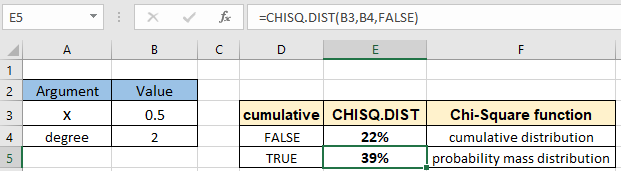In this article, we will learn How to use the CHISQ.DIST function in Excel.

What is Chi square distribution and degree of freedom?

The Chi Square distribution is the distribution of the sum of squared standard normal deviates. For example we sample two variables from a standard normal distribution, square each variable, and sum the squares. What is the probability that the sum of these two squares will be six or higher? Since two variables are taken as sample, the answer can be found using the Chi Square distribution with two degrees of freedom. CHISQ.DIST function can be used to find that the probability of a Chi Square (with 2 degree of freedom) being six or higher is 0.050.

Degree of freedom

Now you must be wondering what is the degree of freedom (also known as df). Mathematically, the degree of freedom(df) of a distribution is equal to the number of standard normal deviations being summed. But how can we understand the concept of degree of freedom? Degree of freedom is the number of independent possibilities in an event. For example if we toss a coin 100 times and say heads occur 48 times and then we can conclude the tails occured 52 times so the degree of freedom is 1. But if we say on a route of traffic lights (generally has 3 color lights) we want to know the probability of red light in any given sample of time. For this the degree of freedom will be 2 as we will require information for at least 2 color lights. See how degrees of freedom (df) affect the chi square density distribution in the different plots shown below.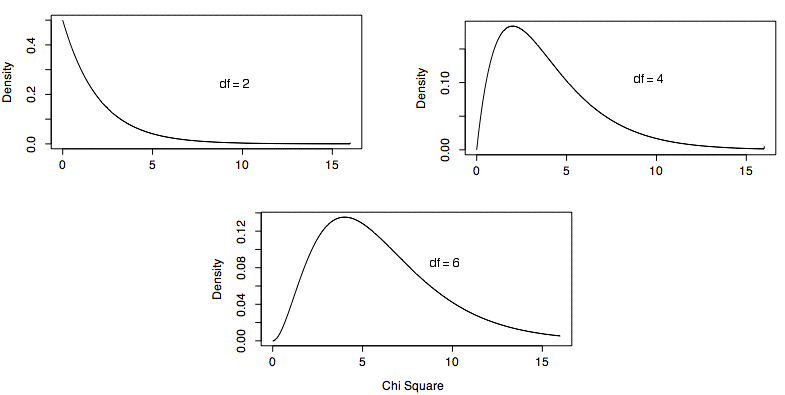Let's learn how to use the CHI SQUARE distribution using the CHISQ.DIST function to calculate the cumulative or probability density for the variable x.

CHISQ.DIST Function in Excel

The CHISQ.DIST function in Excel returns the chi-squared percentage of something across samples, such as the fraction of the day people spend watching television. The function takes variable and degrees of freedom for the distribution along with type of distribution (cdf or pdf).

CHISQ.DIST Function syntax:

 =CHISQ.DIST(x , deg_freedom , cumulative)

x : value at which distribution is evaluated

deg_freedom : degrees of freedom

cumulative : logical value that determines the form of the function. If cumulative is TRUE, CHISQ.DIST returns the cumulative distribution function; if FALSE, it returns the probability density function.

Example :

All of these might be confusing to understand. Let's understand how to use the function using an example. Here we have a sample variable x and degree of freedom. We need to calculate the chi square percentage using the CHISQ.DIST function in Excel

Use the formula:

 =CHISQ.DIST(B3 , B4 , TRUE)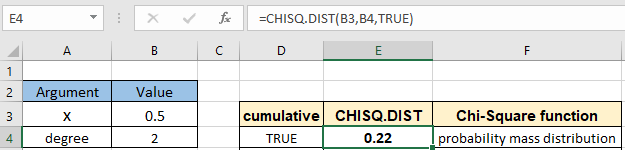The chi square value is in decimal you can convert the value to percentage changing the format of the cell to percentage.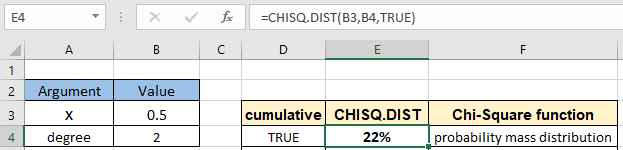The chi square value at least 0.5 comes out to be 22% for the probability mass distribution. Now calculate chi square pdf or probability density value for the same parameters.

Use the formula:

 =CHISQ.DIST(B3 , B4 , FALSE)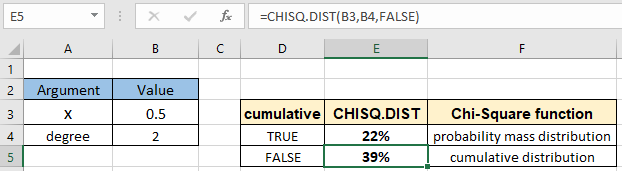The chi square value at exact 0.5 value comes out to be 39% for the cumulative distribution.The Chi Square distribution is very important because many test statistics are distributed as Chi Square. Chi Square distributions are tests of deviations between theoretically expected and observed frequencies.

Here are all the observational notes using the CHISQ.DIST function in Excel
Notes :

1. The function only works with numbers. If any argument other than cumulative is non numeric, the function returns #VALUE! error.
2. The function returns #NUM! Error.
1. If x is negative
2. If the degree of freedom is < 1 or >10^10.
3. The cumulative argument can be used with boolean numbers (0 and 1) or (FALSE or TRUE).
4. Value in decimal and value in percentage id the same value in Excel. Convert the value to percentage, if required.
5. You can feed the arguments to the function directly or using the cell reference as explained in the example.

Hope this article about How to use the CHISQ.DIST function in Excel is explanatory. Find more articles on statistical formulas and related Excel functions here. If you liked our blogs, share it with your friends on Facebook. And also you can follow us on Twitter and Facebook. We would love to hear from you, do let us know how we can improve, complement or innovate our work and make it better for you. Write to us at info@exceltip.com.

Related Articles :

How to use Excel NORM.DIST Function : Calculate the Z score for the normal cumulative distribution for the pre specified values using the NORMDIST function in Excel.

How to use Excel NORM.INV Function : Calculate the inverse of Z score for the normal cumulative distribution for the pre-specified probability values using the NORM.INV function in Excel.

How to Calculate Standard Deviation in Excel : To calculate the standard deviation we have different functions in Excel. The standard deviation is the square root of the variance value but It tells more about the dataset than variance.

Regressions Analysis in Excel : Regression is an Analysis Tool, which we use for analyzing large amounts of data and making forecasts and predictions in Microsoft Excel.

How to Create Standard Deviation Graph : The standard deviation tells how much data is clustered around the average of the data. Learn how to create a standard deviation graph here.

How to use the VAR function in Excel : Calculate the variance for the sample dataset in excel using the VAR function in Excel.

Popular Articles :

How to use the IF Function in Excel : The IF statement in Excel checks the condition and returns a specific value if the condition is TRUE or returns another specific value if FALSE.

How to use the VLOOKUP Function in Excel : This is one of the most used and popular functions of excel that is used to lookup value from different ranges and sheets.

How to use the SUMIF Function in Excel : This is another dashboard essential function. This helps you sum up values on specific conditions.

How to use the COUNTIF Function in Excel : Count values with conditions using this amazing function. You don't need to filter your data to count specific values. Countif function is essential to prepare your dashboard.

Terms and Conditions of use

The applications/code on this site are distributed as is and without warranties or liability. In no event shall the owner of the copyrights, or the authors of the applications/code be liable for any loss of profit, any problems or any damage resulting from the use or evaluation of the applications/code.